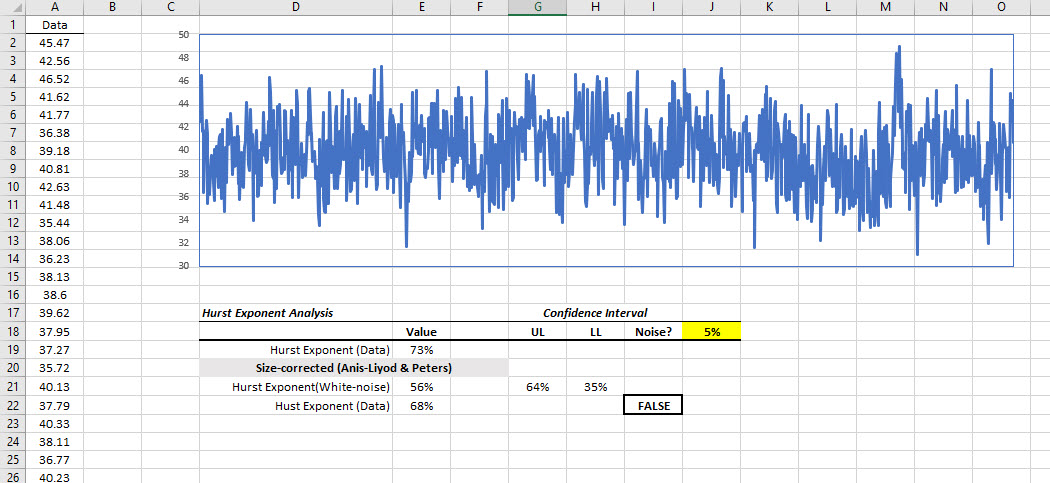# Hurst Exponent, Index, or Parameter

Calculates the Hurst exponent (a measure of persistence or long memory) for time series with more than 96 observations.

## Syntax

Hurst(X, Alpha, Return_type)
X
is the input data sample (a one-dimensional array of cells (e.g. rows or columns)).
Alpha
is the statistical significance of the test (i.e. alpha). If missing or omitted, an alpha value of 5% is assumed.
Return_type
is a number that determines the type of return value: 1 (or missing)=Empirical, 2=(Anis-Lloyd/Peters) Corrected, 3=Theoretical, 4=Upper Limit, 5=Lower Limit.
RETURN_TYPE NUMBER RETURNED
1 or omitted Empirical Hurst exponent
2 Corrected Hurst exponent (Anis-Lloyd/Peters)
3 Theoretical Hurst exponent (Anis-Lloyd/Peters)
4 Upper limit of empirical confidence interval
5 Lower limit of empirical confidence interval

## Remarks

1. The input data series must have at least 96 non-missing values.Otherwise, Hurst function returns #VALUE.
2. The input data series may include missing values (e.g. #N/A, #VALUE!, #NUM!, empty cell), but they will not be included in the calculations.
3. The Hurst exponent, $h$, is defined in terms of the Rescaled Range as follows:

$$E \left [ \frac{R(n)}{S(n)} \right ]=Cn^H \qquad \mathrm{as} \ n \to \infty$$

Where:
• $\left [ \frac{R(n)}{S(n)} \right ]$ is the Rescaled Range.
• $E \left [x \right ]$ is the expected value.
• $n$ is the time of the last observation (e.g. it corresponds to $X_n$ in the input time series data.)
• $h$ is a constant.
4. The Hurst exponent is a measure of autocorrelation (persistence and long memory).

• A value of $0 \lt H \lt 0.5$ indicates a time series with negative autocorrelation (e.g. a decrease between values will probably be followed by another decrease),

• A value of $0.5 \lt H \lt 1$ indicates a time series with positive autocorrelation (e.g. an increase between values will probably be followed by another increase),

• A value of $H=0.5$ indicates a "true random walk," where it is equally likely that a decrease or increase will follow from any particular value (e.g. the time series has no memory of previous values)
5. The Hurst exponent's namesake, Harold Edwin Hurst (1880-1978), was a British hydrologist who researched reservoir capacity along the Nile river.
6. The Rescaled Range is calculated for a time series, $X=X_1,X_2,\dots, X_n$, as follows:

1. Calculate the mean:

$$m=\dfrac{1}{n} \sum_{i=1}^{n} X_i$$
2. Create a mean adjusted series:

$$Y_t=X_{t}-m \qquad \mathrm{for}\ t=1,2, \dots ,n$$
3. Calculate the cumulative deviate series Z:

$$Z_t= \sum_{i=1}^{t} Y_{i} \qquad \mathrm{for}\ t=1,2, \dots ,n$$
4. Create a range series R:

$$R_t = max\left (Z_1, Z_2, \dots, Z_t \right )- min\left (Z_1, Z_2, \dots, Z_t \right ) \qquad \mathrm{for}\ t=1,2, \dots, n$$
5. Create a standard deviation series R:

$$S_{t}= \sqrt{\dfrac{1}{t} \sum_{i=1}^{t}\left ( X_{i} - u \right )^{2}}\qquad \mathrm{for} \ t=1,2, \dots ,n$$

Where:

$h$ is the mean for the time series values $X_1,X_2, \dots, X_t$
6. Calculate the rescaled range series (R/S):

$$\left ( R/S \right )_{t} = \frac{R_{t}}{S_{t}} \qquad \mathrm{for}\ t=1,2, \dots, n$$
7. The Hurst Exponent is estimated by fitting the power-law $E[R(n)/S(n)]=C\times n^H$ to the data. This is done by taking the logarithm of both sides, and fitting a straight line. The slope of the line gives H (i.e. Hurst Exponent Estimate).
8. The method above is known to produce a biased estimate of the power-law exponent, and for small data set, there is a deviation from 0.5 slope (i.e. white-noise). Anis-LIoyd estimated the white-noise theoretical value of the R/S statistics to be as follow: $$\operatorname {E} [R(n)/S(n)]={\begin{cases}{\frac {\Gamma ({\frac {n-1}{2}})}{{\sqrt {\pi }}\Gamma ({\frac {n}{2}})}}\sum \limits _{i=1}^{n-1}{\sqrt {\frac {n-i}{i}}},&{\text{for }}n\leq 340\\{\frac {1}{\sqrt {n{\frac {\pi }{2}}}}}\sum \limits _{i=1}^{n-1}{\sqrt {\frac {n-i}{i}}},&{\text{for }}n>340\end{cases}}$$ Where $\Gamma$ is the Euler Gamma Function
9. No asymptotic distribution theory has been derived for most of the Hurst exponent estimators so far, but an approximate functional forms for Anis-LIoyd corrected R/S estimate is available. For 95% confidence interval, the functional form for confidence interval limits are expressed as follow: $$LL=0.5 - \frac{e^{4.21}}{\ln(M)^{7.33}}$$ $$UL=0.5 + \frac{e^{4.77}}{\ln(M)^{3.10}}$$ Where $M = \log_2(N)$
10. Finally, The Anis-Lloyd corrected R/S Hurst exponent is calculated as 0.5 plus the slope of $R(n)/S(n)-\operatorname {E} [R(n)/S(n)]$

## Illustration## Examples (Hurst Exponent)

Example 1:

1
2
3
4
5
6
7
8
9
10
11
12
13
14
15
16
17
18
19
20
21
22
23
24
25
26
27
28
29
30
A B
Date Data
1/1/2008 #N/A
1/2/2008 -1.28
1/3/2008 0.24
1/4/2008 1.28
1/5/2008 1.20
1/6/2008 1.73
1/7/2008 -2.18
1/8/2008 -0.23
1/9/2008 1.10
1/10/2008 -1.09
1/11/2008 -0.69
1/12/2008 -1.69
1/13/2008 -1.85
1/14/2008 -0.98
1/15/2008 -0.77
1/16/2008 -0.30
1/17/2008 -1.28
1/18/2008 0.24
1/19/2008 1.28
1/20/2008 1.20
1/21/2008 1.73
1/22/2008 -2.18
1/23/2008 -0.23
1/24/2008 1.10
1/25/2008 -1.09
1/26/2008 -0.69
1/27/2008 -1.69
1/28/2008 -1.85
1/29/2008 -0.98

Formula Description (Result)
=HURST($B$2:$B$30,0.05,1) Empirical Hurst exponent (0.583)
=HURST($B$2:$B$30,0.05,2) Corrected Hurst exponent (0.492)

## References

•  A.A.Anis, E.H.Lloyd (1976) The expected value of the adjusted rescaled Hurst range of independent normal summands, Biometrica 63, 283-298.
•  H.E.Hurst (1951) Long-term storage capacity of reservoirs, Transactions of the American Society of Civil Engineers 116, 770-808.
•  E.E.Peters (1994) Fractal Market Analysis, Wiley.
•  R.Weron (2002) Estimating long range dependence: finite sample properties and confidence intervals, Physica A 312, 285-299.
• Hamilton, J .D.; Time Series Analysis , Princeton University Press (1994), ISBN 0-691-04289-6
• Tsay, Ruey S.; Analysis of Financial Time Series John Wiley & SONS. (2005), ISBN 0-471-690740